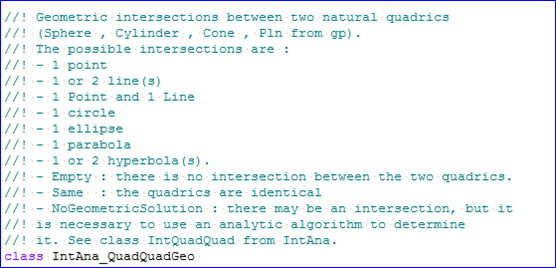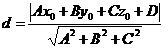# eryar

posts - 432, comments - 579, trackbacks - 0, articles - 0

eryar@163.com

l YIGEDIAN

l YIGEDIANHEYITIAOZHIXIAN

l YUAN

l TUOYUAN

l PAOWUXIAN

l SHUANGQUXIANJIANGYUANMALIECHURUXIA：

```void IntAna_QuadQuadGeo::Perform( const gp_Pln& P
,const gp_Sphere& S)
{
done = Standard_False;
Standard_Real X,Y,Z;
nbint = 0;
// debug JAG : on met typeres = IntAna_Empty par defaut...
typeres = IntAna_Empty;
P.Coefficients(A,B,C,D);
S.Location().Coord(X,Y,Z);
dist = A * X + B * Y + C * Z + D;
// on a une seule solution : le point projection du centre de la sphere
// sur le plan
nbint = 1;
typeres = IntAna_Point;
pt1.SetCoord(X - dist*A, Y - dist*B, Z - dist*C);
}
else if (Abs(dist) < radius) {
// on a un cercle solution
nbint = 1;
typeres = IntAna_Circle;
pt1.SetCoord(X - dist*A, Y - dist*B, Z - dist*C);
dir1 = P.Axis().Direction();
if(P.Direct()==Standard_False) dir1.Reverse();
dir2 = P.Position().XDirection();
}
param2bis=0.0; //-- pour eviter param2bis not used ....
done = Standard_True;
}```

LIJIESHANGSHUDAIMAXUYAOYOUPINGMIANYIBANFANGCHENGDEGAINIANJIDIANDAOPINGMIANDEJULIJISUANGONGSHI：YUANMASHIXIANBUZOURUXIA：

l 取出平面一般方程的系數：ABCD及球心坐標XYZ

l 計算球心到平面的距離dist

l 若距離dist等于球面的半徑，則平面為球面的切平面，只有一個交點；

l 若距離dist小于球面的半徑，則平面與球面的交線為圓；

##### 為了方便大家在移動端也能看到我的博文和討論交流，現已注冊微信公眾號，歡迎大家掃描下方二維碼關注。只有注冊用戶登錄后才能發表評論。 相關文章: 網站導航:                管理